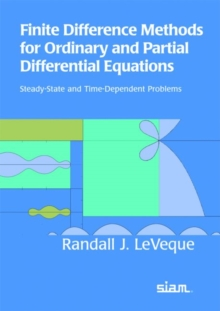Supporting your high street Find out how »
• My Account# Finite Difference Methods for Ordinary and Partial Differential Equations : Steady-state and Time-dependent Problems Paperback

#### Description

This book introduces finite difference methods for both ordinary differential equations (ODEs) and partial differential equations (PDEs) and discusses the similarities and differences between algorithm design and stability analysis for different types of equations.

A unified view of stability theory for ODEs and PDEs is presented, and the interplay between ODE and PDE analysis is stressed.

The text emphasizes standard classical methods, but several newer approaches also are introduced and are described in the context of simple motivating examples. The book is organized into two main sections and a set of appendices.

Part I addresses steady-state boundary value problems, starting with two-point boundary value problems in one dimension, followed by coverage of elliptic problems in two and three dimensions.

It concludes with a chapter on iterative methods for large sparse linear systems that emphasizes systems arising from difference approximations. Part II addresses time-dependent problems, starting with the initial value problem for ODEs, moving on to initial boundary value problems for parabolic and hyperbolic PDEs, and concluding with a chapter on mixed equations combining features of ODEs, parabolic equations, and hyperbolic equations.

The appendices cover concepts pertinent to Parts I and II.

Exercises and student projects, developed in conjunction with this book, are available on the book's webpage along with numerous MATLAB m-files. Readers will gain an understanding of the essential ideas that underlie the development, analysis, and practical use of finite difference methods as well as the key concepts of stability theory, their relation to one another, and their practical implications.

The author provides a foundation from which students can approach more advanced topics and further explore the theory and/or use of finite difference methods according to their interests and needs.

#### Information

• Format: Paperback
• Pages: 354 pages, 120 exercises
• Publisher: Society for Industrial & Applied Mathematics,U.S.
• Publication Date:
• Category: Differential calculus & equations
• ISBN: 9780898716290

£52.00

£45.49

on all orders

###### Pick up orders

from local bookshops

£27.99

£22.75

£99.99

£81.45

£28.00

£23.80

£84.50

£71.83